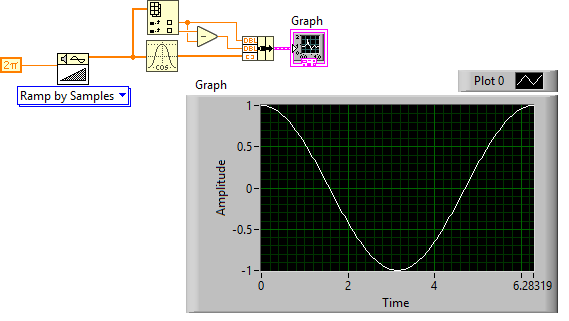# LabVIEW

cancel
Showing results for
Did you mean:

## Doubt in Block Diagram

Good, looking at examples on the Internet about the Clusters, I found the following Block Diagram, but I do not understand the Cluster's function well. My question is if they can help me understand the Block diagram (indicating each part) and why the result of the front panel is obtained.

Thank you !!!

Message 1 of 5
(2,232 Views)

## Re: Doubt in Block Diagram

The constant 20 is the x0 point on the graph.  It's where the signal starts.

The constant 0.5 is the deltaX.  So for every Y value in your cosine array, it is plotted at 0.5 increments along the x axis.

You would learn a lot if you take the free tutorials and simply read the help file for a waveform graph.

aputman
------------------
Heads up! NI has moved LabVIEW to a mandatory SaaS subscription policy, along with a big price increase. Make your voice heard.
Message 2 of 5
(2,220 Views)

## Re: Doubt in Block Diagram

You should also be aware that the input to "cosine" is in radians and calculating it for consecutive integers will give you an alias frequency. You should probably use a x ramp that corresponds to your dx (after picking a reasonable dx).LabVIEW Champion.Message 3 of 5
(2,205 Views)

## Re: Doubt in Block Diagram

And back to the cluster question. Yes a cluster of two numerics and a 1D or 2D numeric array (in exactly that order) is a special data type that is understood by a graph as x0, dx, data. This is a very old legacy structure, predating the waveform data type. I still use it, but be aware that that you might get unexpected results if you also define x0,dx for the x-axis directly. They interfere.LabVIEW Champion.Message 4 of 5
(2,196 Views)

## Re: Doubt in Block Diagram

Here is a typical way to use an x-ramp and get x0,dx from it while also calculating the cosine function. Now the graph x-axis is correct even if you change the ramp (start, end, number of points etc.). No FOR loop needed.LabVIEW Champion.Message 5 of 5
(2,186 Views)